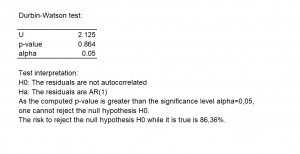# Durbin-Watson-Test

The Durbin-Watson test checks if there is autocorrelation among the residuals of a linear regression. It is available in Excel using the XLSTAT software.## Durbin Watson test definition

Developed by J.Durbin and G.Watson (1950,1951), the Durbin-Watson test is used to detect the autocorrelation in the residuals from a linear regression.

In practice, the errors are often autocorrelated, it leads to undesirable consequences such as sub-optimal least-squares estimates.

Assume that the error terms (epsilon) are stationary and normally distributed with mean 0. The null and alternative hypotheses of the Durbin-Watson test are:

H0: The errors are uncorrelated

H1: The errors are AR(1)

And the test statistic D is:In the context of the Durbin-Watson test, the main problem is the evaluation of the p-values. In XLSTAT the Imhof procedure (1961) is used to solve this problem.

## Results of the Durbin-Watson test in XLSTAT

In XLSTAT, the results of the Durbin-Watson test are the following:

• The tables of descriptive statistics show the simple statistics for the residuals. The number of observations, missing values, the number of non-missing values, the mean and the standard deviation (unbiased) are displayed.

• The value of the statistic D and the p-value of the Durbin-Watson test. A short interpretation is also displayed.### analysieren sie ihre daten mit xlstat

kostenlose 14-tage-testversion

Enthalten ind

Verwandte Funktionen